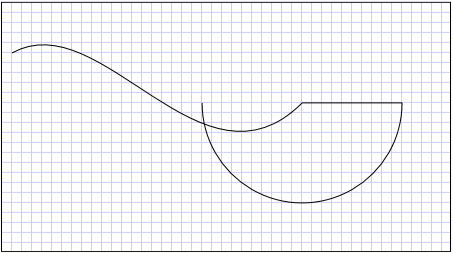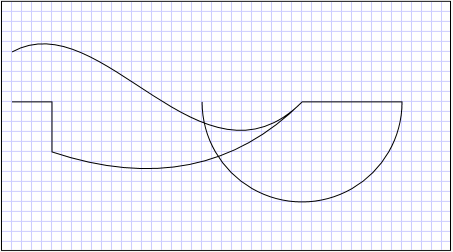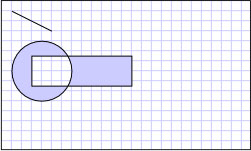# Geometries

Microsoft Silverlight will reach end of support after October 2021. Learn more.

This overview describes how to use Silverlight Geometry objects to define shapes. It also contrasts Geometry objects and Shape elements.

This topic contains the following sections.

• What Is a Geometry?
• Geometries vs. Shapes
• Common Properties That Take a Geometry
• Simple Geometry Types
• Path Geometries
• Composite Geometries
• Related Topics

## What Is a Geometry?

Geometry objects, such as EllipseGeometry, PathGeometry, and GeometryGroup, enable you to describe the geometry of a two-dimensional (2-D) shape. These geometric descriptions have several uses, such defining a shape to paint to the screen or defining clip regions. Geometry objects can be simple (such as rectangles and circles) or composite (created from two or more Geometry objects). More complex geometries can be created by using PathGeometry objects, which enable you to describe arcs and curves.

## Geometries vs. Shapes

The Geometry and Shape classes are similar because they both describe 2-D shapes (compare EllipseGeometry and Ellipse for example), but there are important differences. For example, Shape objects are UIElement objects, but Geometry objects are not. Because they are UIElement objects, Shape objects can render themselves and have Opacity, OpacityMask, and other graphical properties that Geometry objects lack. Although Shape objects are more readily usable than Geometry objects, Geometry objects are more versatile.

One Shape, the Path class, uses a Geometry to describe its contents. By setting the Data property of the Path with a Geometry and setting its Fill and Stroke properties, you can render a Geometry.

## Common Properties That Take a Geometry

The following table lists properties that take a Geometry object.

Type

Property

Path

Data

UIElement

Clip

## Simple Geometry Types

Geometry objects can be grouped into three categories: simple geometries, path geometries, and composite geometries.

Simple geometry classes include LineGeometry, RectangleGeometry, and EllipseGeometry and are used to create basic geometric shapes, such as lines, rectangles, and circles.

• A LineGeometry is defined by specifying the start point and the end point of the line.

• A RectangleGeometry is defined by using a Rect structure that specifies the relative position, height, and width of the rectangle. You can create a rounded rectangle by setting the RadiusX and RadiusY properties.

• An EllipseGeometry is defined by a center point, an x-radius, and a y-radius.

Although these and more complex shapes can be created by using a PathGeometry or by combining geometry objects, the simple geometry classes provide an easy way to produce these basic geometric shapes.

The following example shows how to create and render a LineGeometry. As noted previously, a Geometry object is unable to draw itself, so the example uses a Path shape to render the line. Because a line has no area, setting the Fill property of the Path would have no effect; instead, only the Stroke and StrokeThickness properties are specified.

``````<Canvas Width="200" Height="200">
<Path Stroke="Black" StrokeThickness="1" >
<Path.Data>
<LineGeometry StartPoint="10,20" EndPoint="100,130" />
</Path.Data>
</Path>
</Canvas>
``````

The following illustration shows the output from the example.

A LineGeometry drawn from (10,20) to (100,130)The next example shows how to create and render an EllipseGeometry. The example sets the Center of the EllipseGeometry to the point 50,50 and the x-radius and the y-radius to 50, which creates a circle with a diameter of 100. The interior of the ellipse is painted by assigning a value to the Path element's Fill property, in this case Gold.

``````<Canvas>
<Path Fill="Gold" Stroke="Black" StrokeThickness="1">
<Path.Data>
</Path.Data>
</Path>
</Canvas>
``````

The following illustration shows the output from the example.

An EllipseGeometry drawn at (50,50)The following example shows how to create and render a RectangleGeometry. The position and the dimensions of the rectangle are defined by a Rect structure. The position is 50,50 and the height and width are both 25, which creates a square.

``````<Canvas>
<Path Fill="LemonChiffon" Stroke="Black" StrokeThickness="1">
<Path.Data>
<RectangleGeometry Rect="50,50,25,25" />
</Path.Data>
</Path>
</Canvas>
``````

The following illustration shows the output of the example.

A RectangleGeometry drawn at (50,50)The following example shows how to use an EllipseGeometry as the clip region for an image. An Image object is defined with a Width of 200 and a Height of 150. An EllipseGeometry with a RadiusX value of 100, a RadiusY value of 75, and a Center value of 100,75 is set to the Clip property of the image. Only the part of the image that is within the area of the ellipse is displayed.

``````<Grid x:Name="LayoutRoot" Background="White">
<Image Source="Water_lilies.jpg"
Width="200" Height="150">
<Image.Clip>
</Image.Clip>
</Image>
</Grid>
``````

The following illustration shows the output from the example.

An EllipseGeometry used to clip an Image## Path Geometries

The PathGeometry object and the geometry mini-language provide the means to describe multiple complex figures that are composed of arcs, curves, and lines.

At the heart of a PathGeometry is a collection of PathFigure objects, so named because each figure describes a discrete shape in the PathGeometry. Each PathFigure is itself composed of one or more PathSegment objects, each of which describes a segment of the figure. The following table lists the different types of segments.

Segment type

Description

ArcSegment

Creates an elliptical arc between two points.

BezierSegment

Creates a cubic Bezier curve between two points.

LineSegment

Creates a line between two points.

PolyBezierSegment

Creates a series of cubic Bezier curves.

PolyLineSegment

Creates a series of lines.

Creates a series of quadratic Bezier curves.

Creates a quadratic Bezier curve.

The segments within a PathFigure are combined into a single geometric shape, with the end point of each segment becoming the start point of the next segment. The StartPoint property of a PathFigure specifies the point from which the first segment is drawn. Each subsequent segment starts at the end point of the previous segment. For example, a vertical line from 10,50 to 10,150 can be defined by setting the StartPoint property to 10,50 and creating a LineSegment with a Point property setting of 10,150.

The following example creates a simple PathGeometry that contains a single PathFigure with a LineSegment and displays it by using a Path element. The PathFigure object's StartPoint is set to 10,20, and a LineSegment is defined with an end point of 100,130.

``````<Canvas>
<Path Stroke="Black" StrokeThickness="1">
<Path.Data>
<PathGeometry>
<PathGeometry.Figures>
<PathFigure StartPoint="10,20">
<PathFigure.Segments>
<LineSegment Point="100,130"/>
</PathFigure.Segments>
</PathFigure>
</PathGeometry.Figures>
</PathGeometry>
</Path.Data>
</Path>
</Canvas>
``````

The following illustration shows the PathGeometry created by this example.

A PathGeometry that contains a single LineSegmentIt is worth contrasting this example with the preceding LineGeometry example. The syntax used for a PathGeometry is much more verbose than that used for a simple LineGeometry, and it may make more sense to use the LineGeometry class in this case, but the verbose syntax of the PathGeometry allows for extremely intricate and complex geometric regions.

Geometries of greater complexities can be created by using a combination of PathSegment objects.

The next example uses a BezierSegment, a LineSegment, and an ArcSegment to create a shape.

• The example first creates a cubic Bezier curve by defining four points: a start point, which is the end point of the previous segment, an end point (Point3), and two control points (Point1 and Point2). The two control points of a cubic Bezier curve behave like magnets, attracting portions of what would otherwise be a straight line toward themselves, producing a curve. The first control point, Point1, affects the beginning portion of the curve; the second control point, Point2, affects the ending portion of the curve.

• The example then adds a LineSegment, which is drawn between the end point of the preceding BezierSegment to the point specified by its LineSegment property.

• The example then adds an ArcSegment, which is drawn from the end point of the preceding LineSegment to the point specified by its Point property. The example also specifies the arc's x-radius and y-radius (Size), a rotation angle (RotationAngle), a flag that indicates how large the angle of the resulting arc should be (IsLargeArc), and a value that indicates the direction in which the arc is drawn (SweepDirection).

``````<Canvas>
<Path Stroke="Black" StrokeThickness="1" >
<Path.Data>
<PathGeometry>
<PathGeometry.Figures>
<PathFigure StartPoint="10,50">
<PathFigure.Segments>
<BezierSegment Point1="100,0"
Point2="200,200"
Point3="300,100"/>
<LineSegment Point="400,100" />
<ArcSegment Size="50,50" RotationAngle="45"
IsLargeArc="True" SweepDirection="Clockwise"
Point="200,100"/>
</PathFigure.Segments>
</PathFigure>
</PathGeometry.Figures>
</PathGeometry>
</Path.Data>
</Path>
</Canvas>
``````

The following illustration shows the shape created by this example.

A PathGeometryEven more complex geometries can be created by using multiple PathFigure objects within a PathGeometry.

The following example creates a PathGeometry with two PathFigure objects, each of which contains multiple PathSegment objects. The PathFigure from the previous example and a PathFigure with a PolyLineSegment and a QuadraticBezierSegment are used. A PolyLineSegment is defined with a list of points, and the QuadraticBezierSegment is defined with a control point and an end point.

``````<Canvas>
<Path Stroke="Black" StrokeThickness="1" >
<Path.Data>
<PathGeometry>
<PathGeometry.Figures>
<PathFigure StartPoint="10,50">
<PathFigure.Segments>
<BezierSegment Point1="100,0"
Point2="200,200"
Point3="300,100"/>
<LineSegment Point="400,100" />
<ArcSegment Size="50,50" RotationAngle="45"
IsLargeArc="True" SweepDirection="Clockwise"
Point="200,100"/>
</PathFigure.Segments>
</PathFigure>

<PathFigure StartPoint="10,100">
<PathFigure.Segments>
<PolyLineSegment Points="50,100 50,150" />
</PathFigure.Segments>
</PathFigure>
</PathGeometry.Figures>
</PathGeometry>
</Path.Data>
</Path>
</Canvas>
``````

The following illustration shows the shape created by this example.

A PathGeometry with multiple figures### Path Markup Syntax

Silverlight Geometry objects support a XAML attribute syntax that uses a special series of move and draw commands. For more information, see Path Markup Syntax.

## Composite Geometries

Composite geometry objects can be created by using a GeometryGroup object. GeometryGroup creates an amalgamation of the Geometry objects it contains without combining their area. Any number of Geometry objects can be added to a GeometryGroup. The following example uses a GeometryGroup to create a composite Geometry.

``````<Canvas>
<Path Stroke="Black" StrokeThickness="1" Fill="#CCCCFF">
<Path.Data>

<!-- Creates a composite shape from three geometries. -->
<GeometryGroup FillRule="EvenOdd">
<LineGeometry StartPoint="10,10" EndPoint="50,30" />
<RectangleGeometry Rect="30,55 100 30" />
</GeometryGroup>
</Path.Data>
</Path>
</Canvas>
``````

The following illustration shows the output of the example.

A composite Geometry